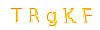## javascript 内置对象 math,global

1.Global对象
2.Math对象

ECMA-262对内置对象的定义是：“由ECMAScript实现提供的、不依赖宿主环境的对象，这些对象在ECMAScript程序执行之前就已经存在了。”意思就是说，开发人员不必显示地实例化内置对象；因为它们已经实例化了。ECMA-262只定义了两个内置对象：Global和Math。

Global(全局)对象是ECMAScript中一个特别的对象，因为这个对象是不存在的。在ECMAScript中不属于任何其他对象的属性和方法，都属于它的属性和方法。所以，事实上，并不存在全局变量和全局函数；所有在全局作用域定义的变量和函数，都是Global对象的属性和方法。
PS：因为ECMAScript没有定义怎么调用Global对象，所以，Global.属性或者Global.方法()都是无效的。(Web浏览器将Global作为window对象的一部分加以实现)

Global对象有一些内置的属性和方法：

1.URI编码方法
URI编码可以对链接进行编码，以便发送给浏览器。它们采用特殊的UTF-8编码替换所有无效字符，从而让浏览器能够接受和理解。
encodeURI()不会对本身属于URI的特殊字符进行编码，例如冒号、正斜杠、问号和#号；而encodeURIComponent()则会对它发现的任何非标准字符进行编码

```var box = '//Lee李';

var box = '//Lee李';
```

PS：因为encodeURIComponent()编码比encodeURI()编码来的更加彻底，一般来说encodeURIComponent()使用频率要高一些。

```var box = '//Lee李';

var box = '//Lee李';
```

PS：URI方法如上所述的四种，用于代替已经被ECMA-262第3版废弃的escape()和unescape()方法。URI方法能够编码所有的Unicode字符，而原来的只能正确地编码ASCII字符。所以建议不要再使用escape()和unescape()方法。

2.eval()方法
eval()方法主要担当一个字符串解析器的作用，他只接受一个参数，而这个参数就是要执行的JavaScript代码的字符串。
```eval('var box = 100');						//解析了字符串代码

eval('function box() {return 123}');			//函数也可以
```

eval()方法的功能非常强大，但也非常危险。因此使用的时候必须极为谨慎。特别是在用户输入数据的情况下，非常有可能导致程序的安全性，比如代码注入等等。

3.Global对象属性
Global对象包含了一些属性：undefined、NaN、Object、Array、Function等等。

4.window对象

ECMAScript还为保存数学公式和信息提供了一个对象，即Math对象。与我们在JavaScript直接编写计算功能相比，Math对象提供的计算功能执行起来要快得多。

1.Math对象的属性
Math对象包含的属性大都是数学计算中可能会用到的一些特殊值。

Math.E 自然对数的底数，即常量e的值
Math.LN10 10的自然对数
Math.LN2 2的自然对数
Math.LOG2E 以2为底e的对数
Math.LOG10E 以10为底e的对数
Math.PI ∏的值
Math.SQRT1_2 1/2的平方根
Math.SQRT2 2的平方根

2.min()和max()方法
Math.min()用于确定一组数值中的最小值。Math.max()用于确定一组数值中的最大值。

3.舍入方法
Math.ceil()执行向上舍入，即它总是将数值向上舍入为最接近的整数；
Math.floor()执行向下舍入，即它总是将数值向下舍入为最接近的整数；
Math.round()执行标准舍入，即它总是将数值四舍五入为最接近的整数；

4.random()方法
Math.random()方法返回介于0到1之间一个随机数，不包括0和1。如果想大于这个范围的话，可以套用一下公式：

```alert(Math.floor(Math.random() * 10 + 1));		//随机产生1-10之间的任意数
for (var i = 0; i<10;i ++) {
document.write(Math.floor(Math.random() * 10 + 5));		//5-14之间的任意数
document.write('');
}
```

```function selectFrom(lower, upper) {
var sum = upper - lower + 1;							//总数-第一个数+1
return Math.floor(Math.random() * sum + lower);
}

for (var i=0 ;i<10;i++) {
document.write(selectFrom(5,10));					//直接传递范围即可
document.write('');
}
```

5.其他方法

Math.abs(num) 返回num的绝对值
Math.exp(num) 返回Math.E的num次幂
Math.log(num) 返回num的自然对数
Math.pow(num,power) 返回num的power次幂
Math.sqrt(num) 返回num的平方根
Math.acos(x) 返回x的反余弦值
Math.asin(x) 返回x的反正弦值
Math.atan(x) 返回x的反正切值
Math.atan2(y,x) 返回y/x的反正切值
Math.cos(x) 返回x的余弦值
Math.sin(x) 返回x的正弦值
Math.tan(x) 返回x的正切值

评论 ( What Do You Think )
 名称 邮箱 网址 评论 验证•微信公众号
•我的微信

1、一号门博客CMS,由Python, MySQL, Nginx, Wsgi 强力驱动

2、部分文章来源于互联网, 若有侵权, 联系邮箱：summer@yihaomen.com, 同时欢迎大家注册用户，主动发布文章.

3、鄂ICP备14001754号-3, 鄂公网安备 42280202422812号